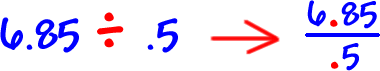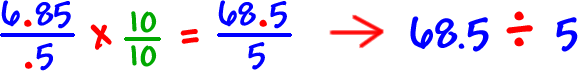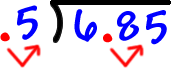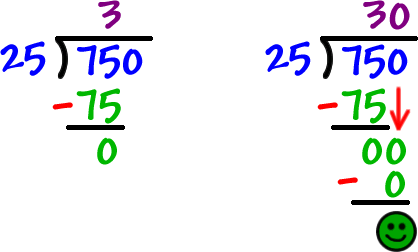OK, so WHY does this work?

Let's write the last problem as a fraction:Can we multiply this fraction by 1 and not change its value?  Yep!

How about if we multiply by a magic 1?YEP!So, just moving both decimals to the rightis the same as multiplying by a magic 1!

Here's another one:

Divide 7.5 by .25

Set it up...Hey, there aren't even any decimals left in this one!

Go for it: